# Motion in a Plane | Definition, Formulas, Types – Motion in a Plane (Projectile and Circular Motion)

Motion in a Plane Physics:
Motion in plane is called as motion in two dimensions, e.g. projectile motion, circular motion. For the analysis of such motion our reference will be made of an origin and two co-ordinate axes X and Y.

We are giving a detailed and clear sheet on all Physics Notes that are very useful to understand the Basic Physics Concepts.

## Motion in a Plane | Definition, Formulas, Types – Motion in a Plane (Projectile and Circular Motion)

Terms Related to Motion in Plane
Few terms related to motion in plane are given below

1. Position Vector Definition:
A vector that extends from a reference point to the point at which particle is located is called position vector.Position vector is given by r = $$\hat{x} \hat{\mathbf{i}}+y \hat{\mathbf{j}}$$
Direction of this position vector r is given by the angle θ with X-axis,
where, tan θ = $$\frac{y}{x}$$
In three dimensions, the position vector is represented as
$$\mathbf{r}=x \hat{\mathbf{i}}+y \hat{\mathbf{j}}+z \hat{\mathbf{k}}$$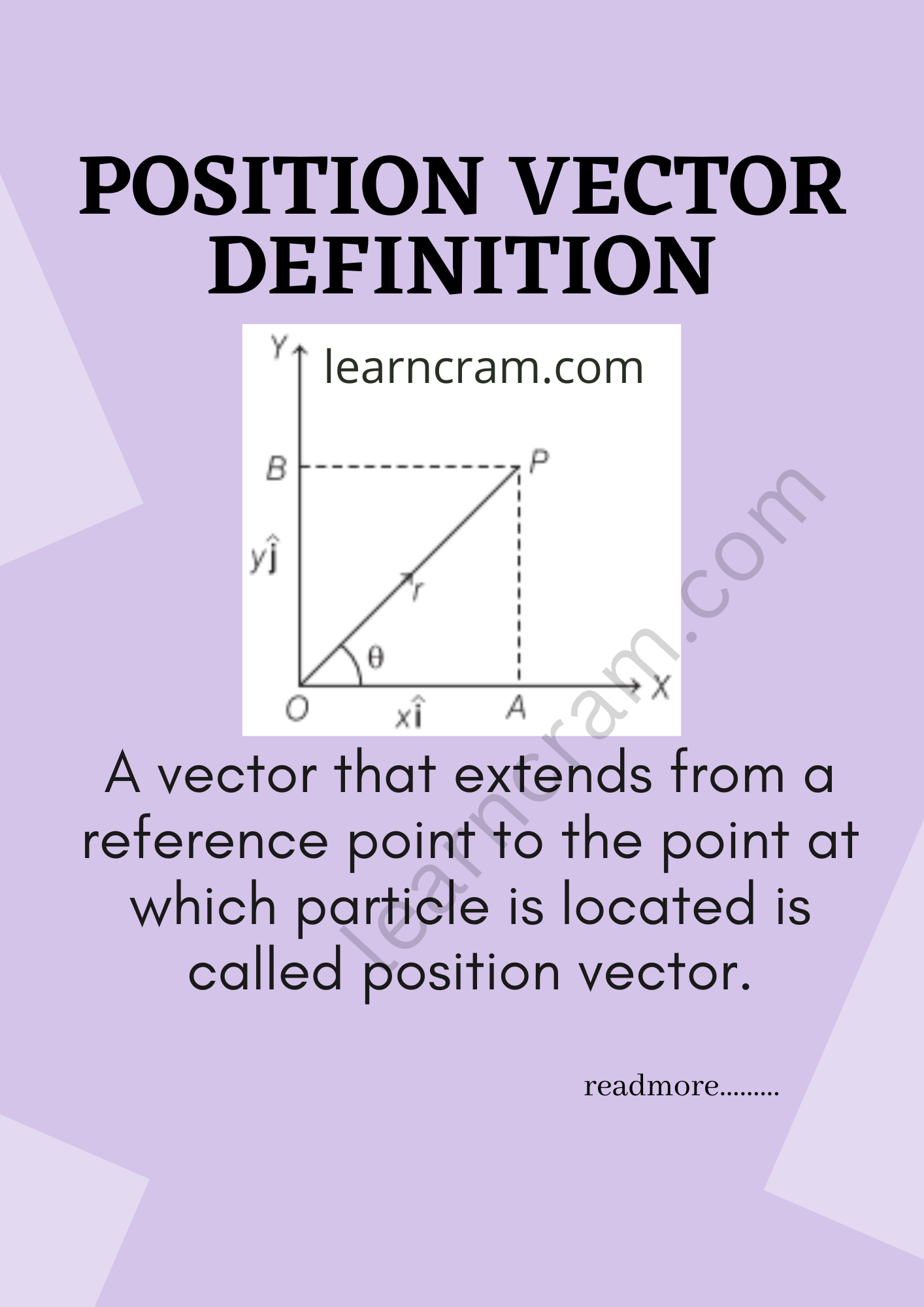2. Displacement Vector Definition:
The displacement vector is a vector which gives the position of a point with reference to a point other than the origin of the co-ordinate system.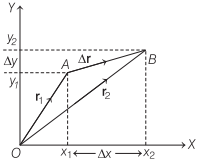Magnitude of displacement vector
\begin{aligned} |\Delta \mathbf{r}| &=\sqrt{(\Delta x)^{2}+(\Delta y)^{2}} \\ &=\sqrt{\left(x_{2}-x_{1}\right)^{2}+\left(y_{2}-y_{1}\right)^{2}} \end{aligned}

Direction of the displacement vector Ar is given by
tan θ = $$\frac{\Delta y}{\Delta x}$$

In three dimensions, the displacement can be represented as
$$\Delta \mathbf{r}=\left(x_{2}-x_{1}\right) \hat{\mathbf{i}}+\left(y_{2}-y_{1}\right) \hat{\mathbf{j}}+\left(z_{2}-z_{1}\right) \hat{\mathbf{k}}$$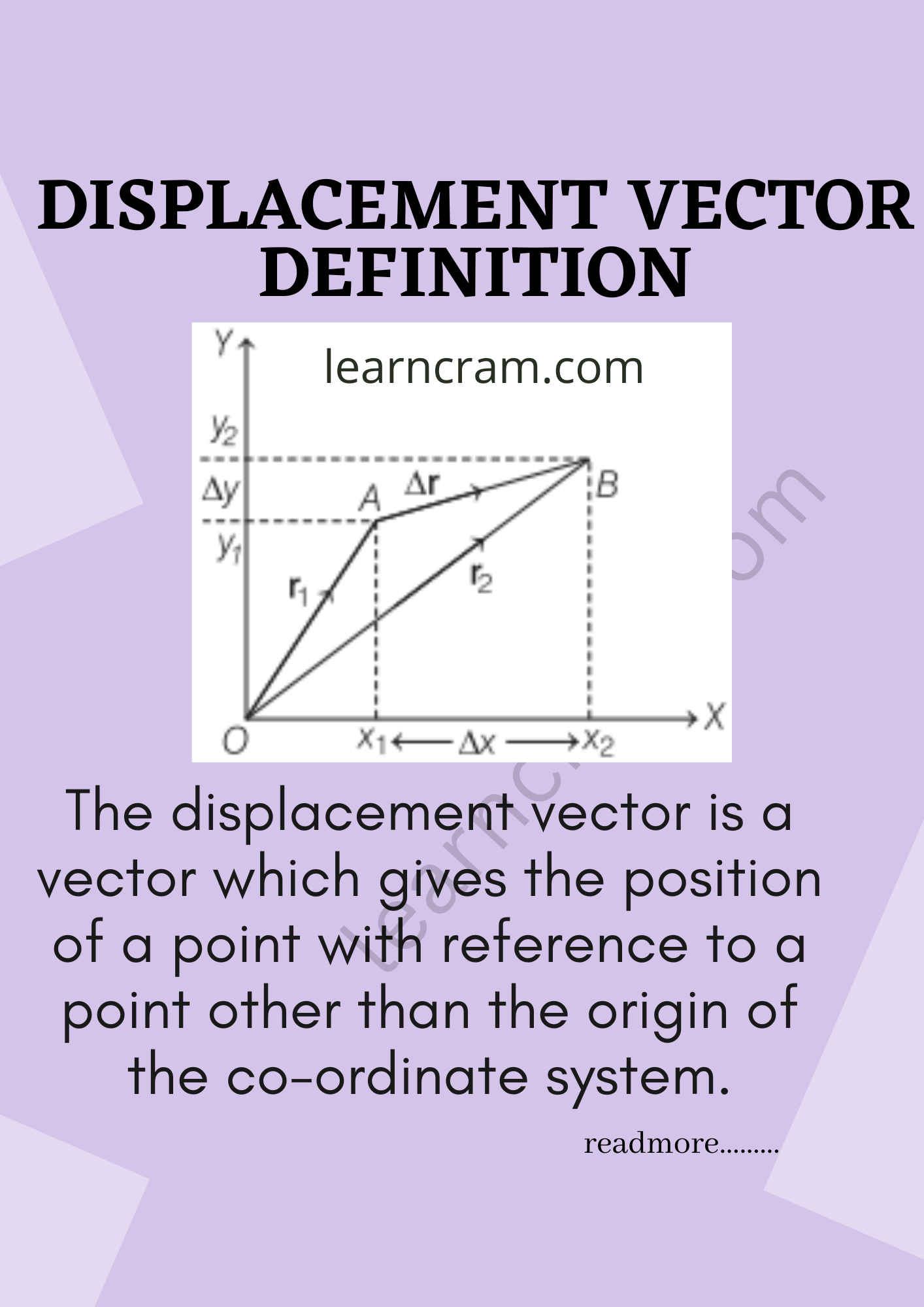3. Velocity Vector Definition:
Velocity of an object in motion is defined as the ratio of displacement and the corresponding time interval taken by the object.
(i) Average Velocity Definition:
It is defined as the ratio of the displacement and the corresponding time interval.Average velocity, $$v_{\mathrm{av}}=\frac{\Delta r}{\Delta t}=\frac{r_{2}-r_{1}}{t_{2}-t_{1}}$$
Average velocity can be expressed in the component forms as
$$v_{\mathrm{av}}=\frac{\Delta x}{\Delta t} \hat{\mathbf{i}}+\frac{\Delta y}{\Delta t} \hat{\mathbf{j}}=\Delta v_{x} \hat{\mathbf{i}}+\Delta v_{y} \hat{\mathbf{j}}$$

The magnitude of uav is given by
tan θ = $$\frac{\Delta v_{y}}{\Delta v_{x}}$$

(ii) Instantaneous Velocity Definition:
The velocity at an instant of time (t) is known as instantaneous velocity.
Instantaneous velocity,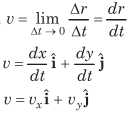Magnitude of instantaneous velocity
$$|v|=\sqrt{v_{x}^{2}+v_{y}^{2}}$$
Direction of v is given by
tan θ = $$\left(\frac{v_{y}}{v_{x}}\right)$$

4. Acceleration Vector:
It is defined as the rate of change of velocity.
(i) Average Acceleration Definition:
It is defined as the change in velocity (Δv) divided by the corresponding time interval (Δt).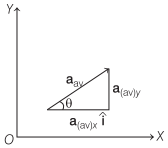Average acceleration,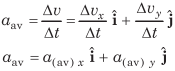Magnitude of average acceleration is given by
$$\left|a_{\mathrm{av}}\right|=\sqrt{\left(a_{(\mathrm{av})} x\right)^{2}+\left(a_{(\mathrm{av})} y^{2}\right)^{2}}$$
Angle θ made by average acceleration with X-axis is
tan θ = $$\frac{a_{y}}{a_{x}}$$

(ii) Instantaneous Acceleration Definition:
It is defined as the limiting value of the average acceleration as the time interval approaches to zero.
Instantaneous acceleration,
a = $$\lim _{\Delta t \rightarrow 0} \frac{d v}{d t}$$
a = $$a_{x} \hat{\mathbf{i}}+a_{y} \hat{\mathbf{j}}$$
If acceleration a makes an angle θ with X-axis
then,
tan θ = $$\left(\frac{a_{y}}{a_{x}}\right)$$

Motion in Plane with Uniform Acceleration
A body is said to be moving with uniform acceleration, if its velocity vector suffers the same change in the same interval of time however small.
According to definition of average acceleration, we have
$$a=\frac{v-v_{0}}{t-0}=\frac{v-v_{0}}{t}$$
v = v0 + at
In terms of rectangular component, we can express it as
vx = v0x + axt
and
vy = v0y + ayt

Path of Particle Under Constant Acceleration
Now, we can also find the position vector (r). Let r0 and r be the position vectors of the particle at time t = 0 and t = t and their velocities at these instants be v0 and v respectively. Then, the average velocity is given by
$$\mathbf{v}_{\mathrm{av}}=\frac{\mathbf{v}_{0}+\mathbf{v}}{2}$$
Displacement is the product of average velocity and time interval. It is expressed asIn terms of rectangular components, we have
$$x \hat{\mathbf{i}}+y \hat{\mathbf{j}}=x_{0} \hat{\mathbf{i}}+y \hat{\mathbf{j}}+\left(v_{0 x} \hat{\mathbf{i}}+v_{0, j} \hat{\mathbf{j}}\right) t+\frac{1}{2}\left(a_{x} \hat{\mathbf{i}}+a_{y} \hat{\mathbf{j}}\right) t^{2}$$

Now, equating the coefficients of $$\hat{\mathbf{i}} \text { and } \hat{\mathbf{j}},$$
x = x0 + v0xt + $$\frac{1}{2}$$axt² ……………. along x-axis
and
y = y0 + v0yt + $$\frac{1}{2}$$ayt² ……………. along y-axis

Note: Motion in a plane (two-dimensional motion) can be treated as two separate simultaneous one-dimensional motions with constant acceleration along two perpendicular directions.

Motion in a Plane (Projectile and Circular Motion):
In this chapter or under this topic, we are going to come across the motion of the object when it is thrown from one end to another end. This practice is said to be projection. Also, when an object is moved in a circular motion, then the equation of the motion is derived here. We will learn here about centripetal force and centripetal acceleration in detail with formulas. Also learn the force applied in everyday life motion of the particle in a vertical circle.